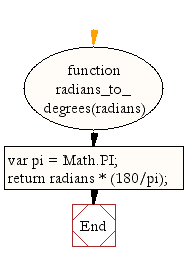# JavaScript: Convert radians to degrees

## JavaScript Math: Exercise-34 with Solution

Write a JavaScript function to convert radians to degrees.

45

Sample Solution-1:

JavaScript Code:

``````function radians_to_degrees(radians)
{
var pi = Math.PI;
}

```
```

Sample Output:

```45
```

Pictorial Presentation:Flowchart:Sample Solution-2:

JavaScript Code:

``````const radians_to_degrees = rad => (rad * 180.0) / Math.PI;
```
```

Sample Output:

```90
45
```

Live Demo:

See the Pen javascript-math-exercise-34 by w3resource (@w3resource) on CodePen.

Improve this sample solution and post your code through Disqus

What is the difficulty level of this exercise?

Test your Programming skills with w3resource's quiz.

﻿